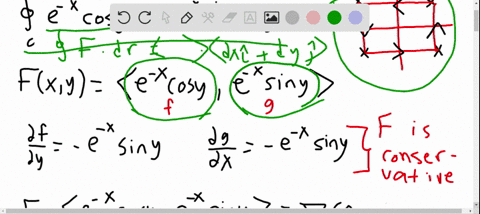🎉 The Study-to-Win Winning Ticket number has been announced! Go to your Tickets dashboard to see if you won! 🎉View Winning Ticket### Evaluate the following line integrals using a met…

06:15Problem 53

# Evaluate the following line integrals using a method of your choice.$\oint_{C} \mathbf{F} \cdot d \mathbf{r},$ where $\mathbf{F}=\left\langle 2 x y+z^{2}, x^{2}, 2 x z\right\rangle$ and $C$ is the circle$\mathbf{r}(t)=\langle 3 \cos t, 4 \cos t, 5 \sin t\rangle,$ for $0 \leq t \leq 2 \pi$

## Discussion

You must be signed in to discuss.